Miscellaneous

Chapter 7 Class 11 Binomial Theorem
Serial order wise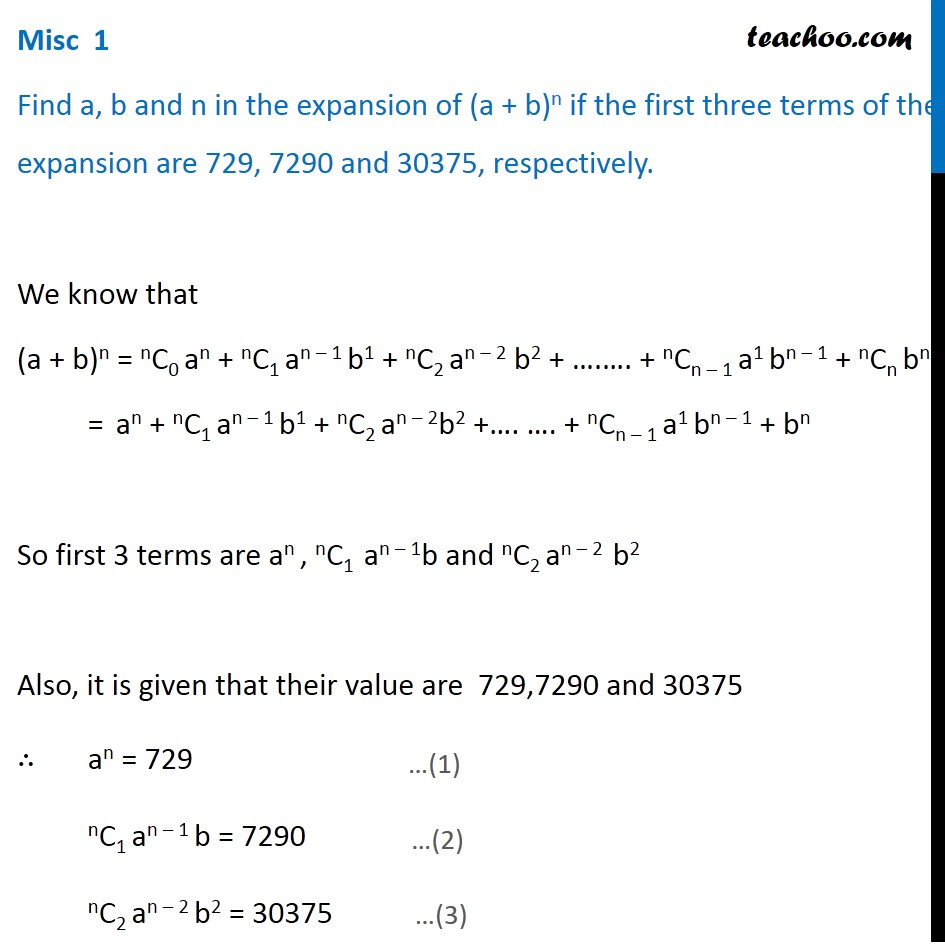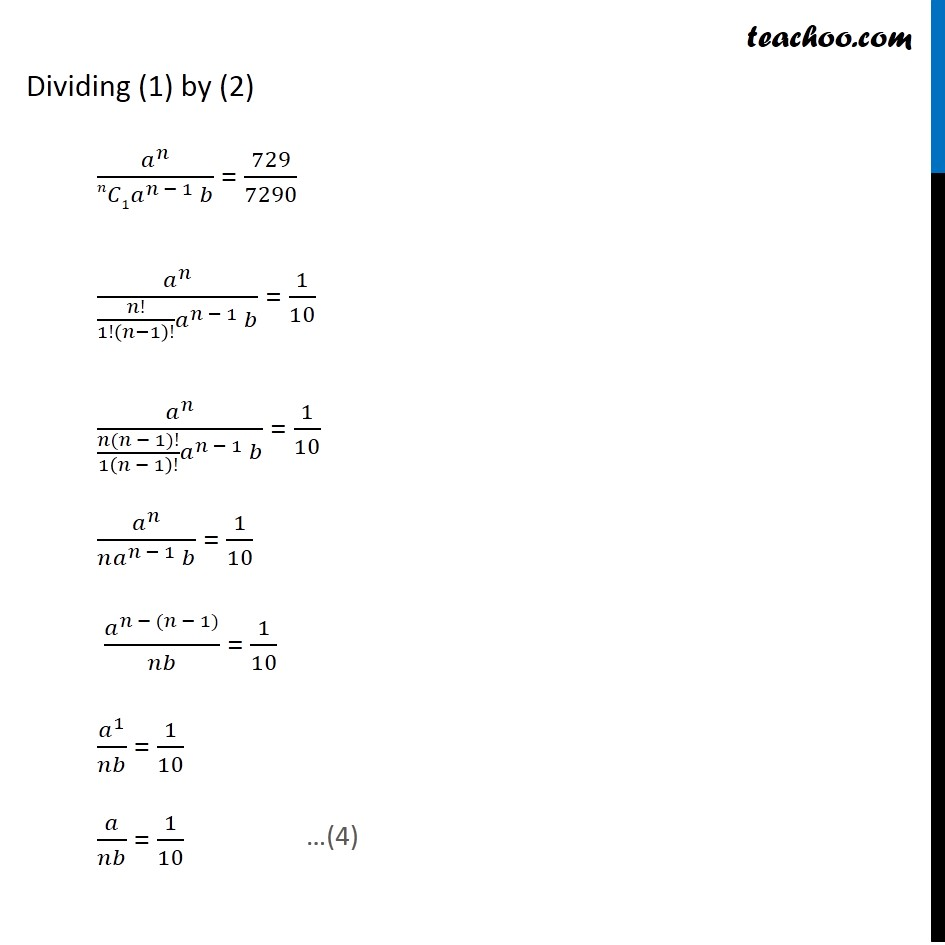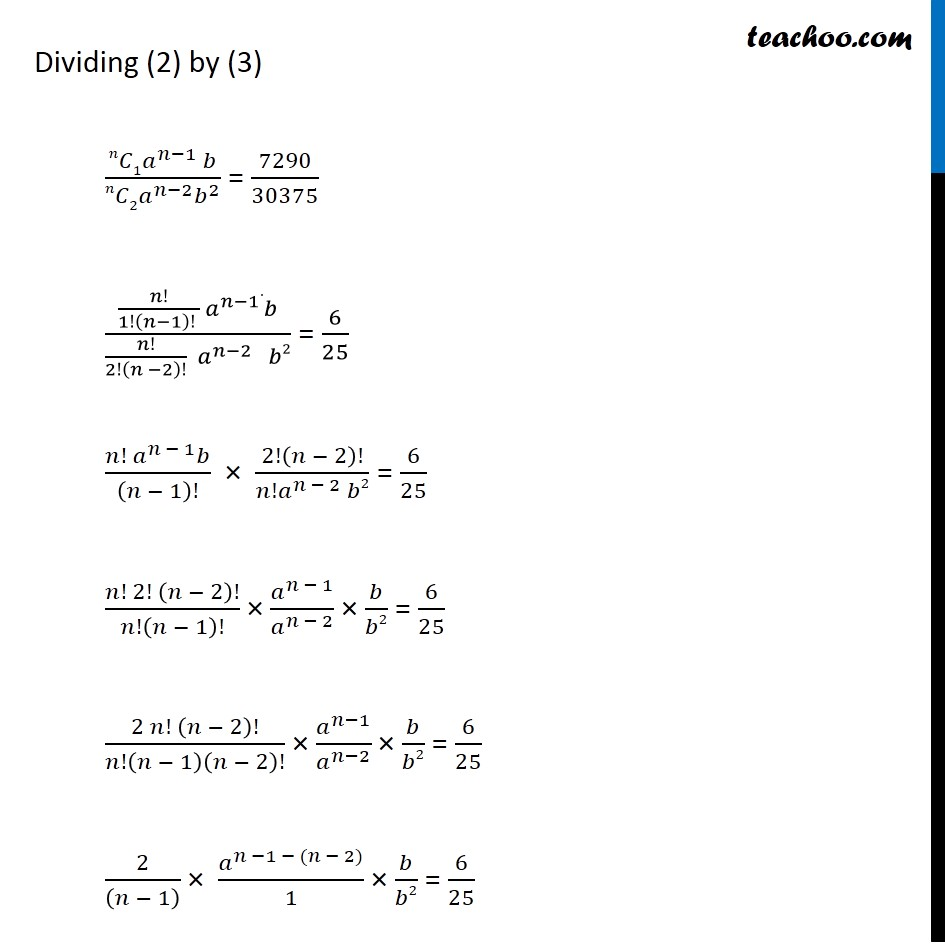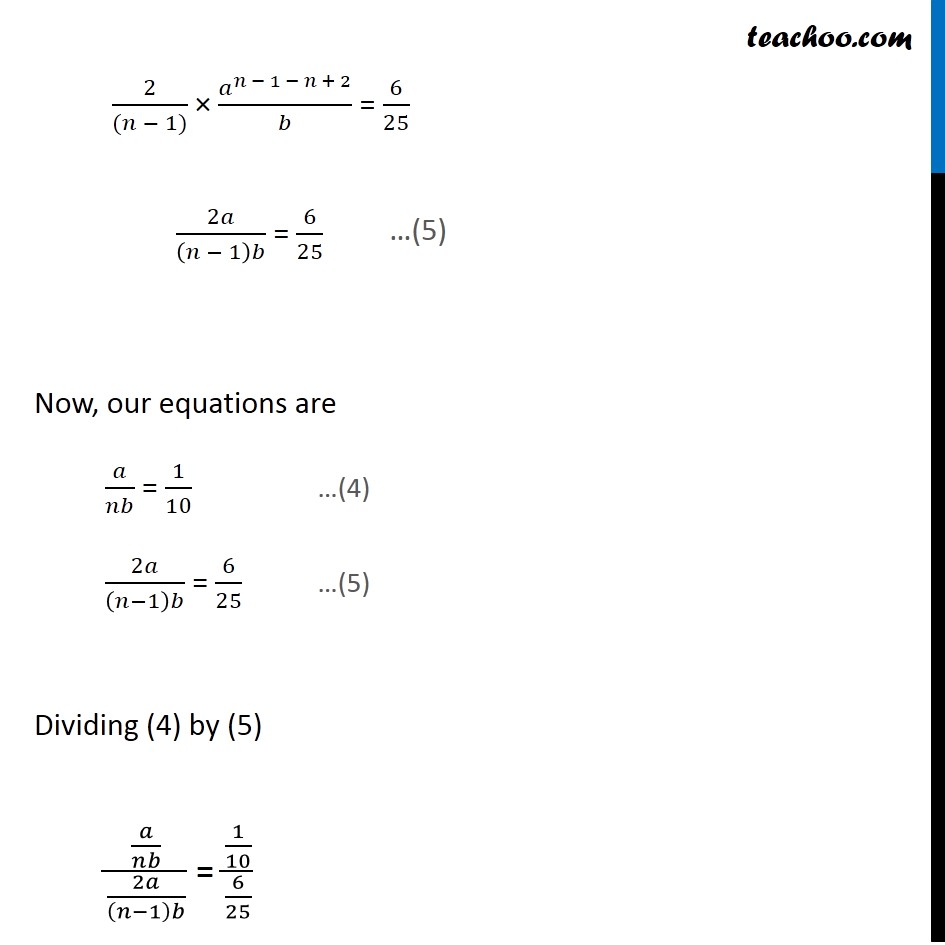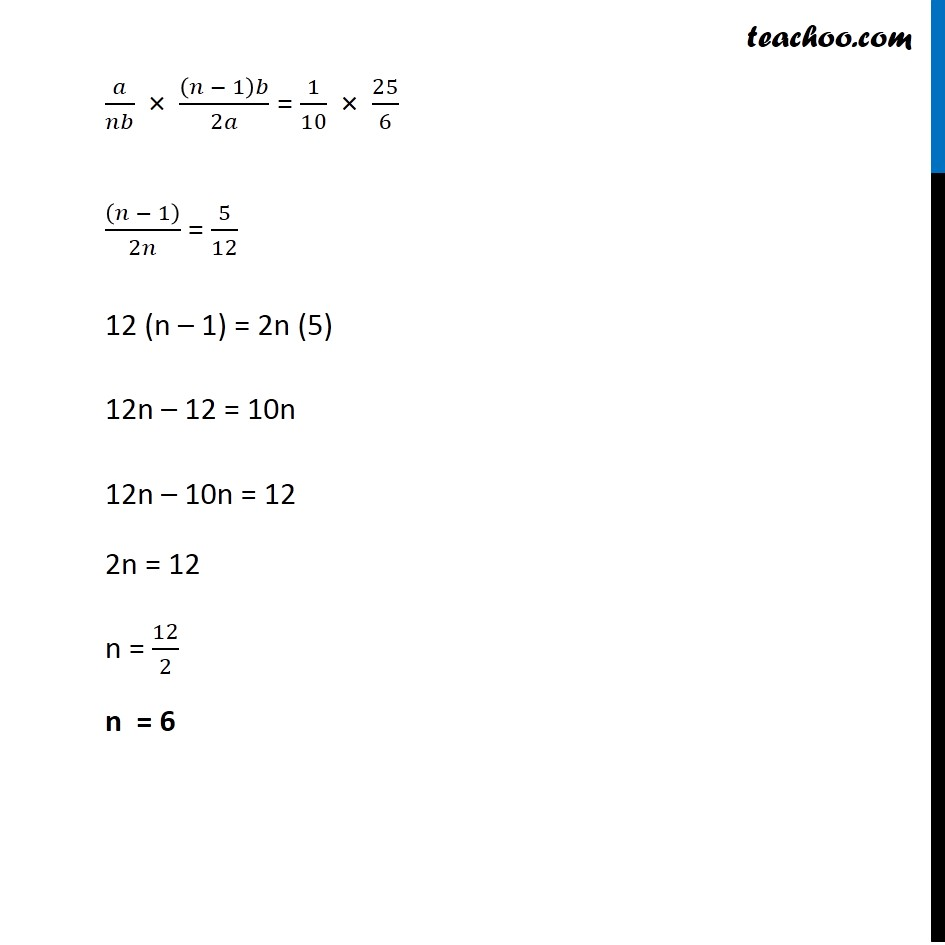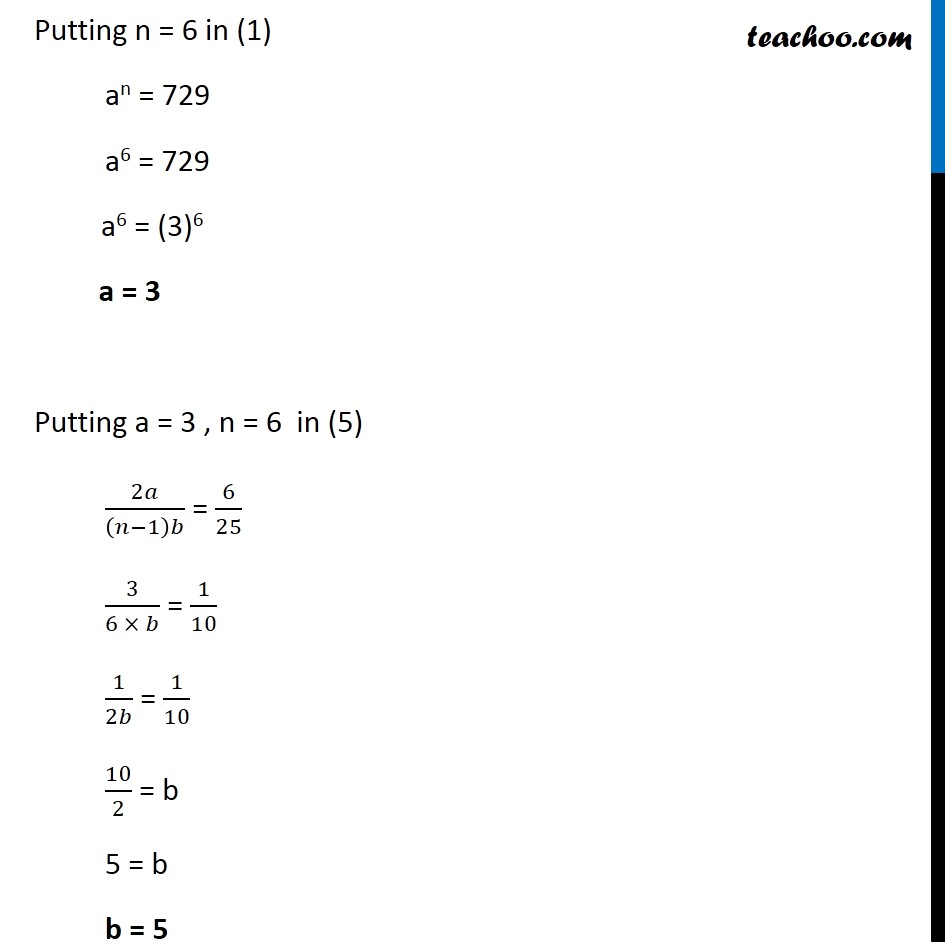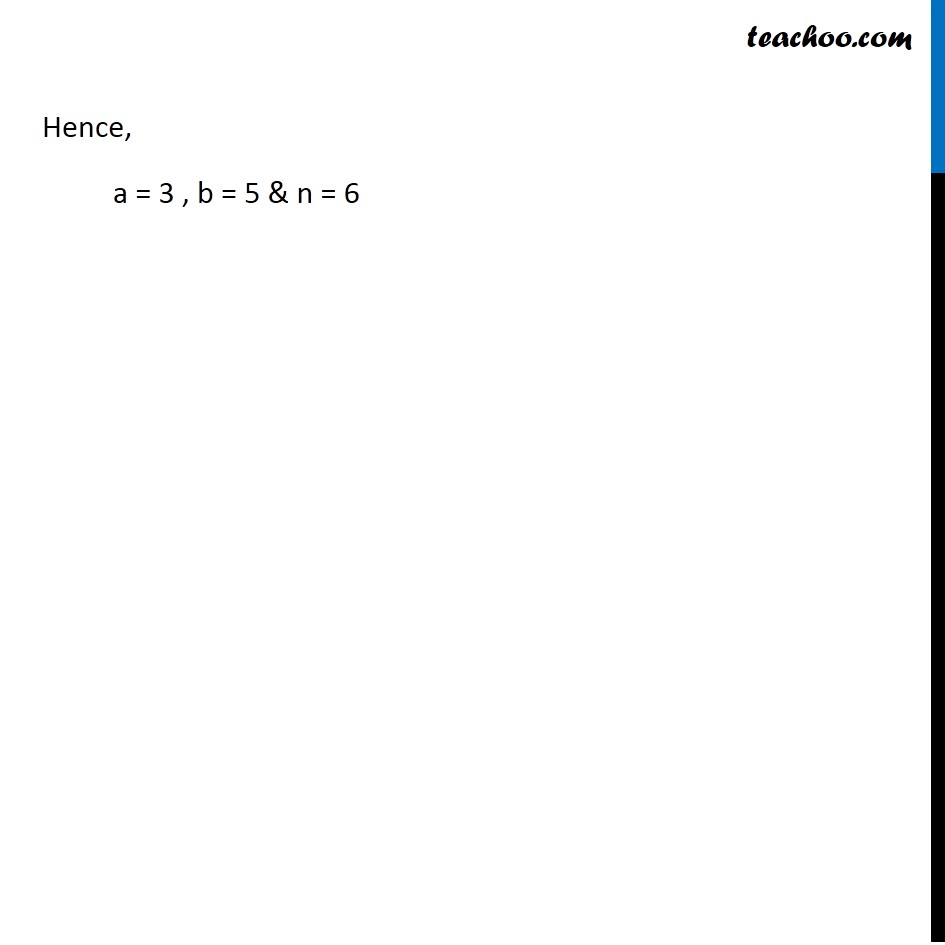Learn in your speed, with individual attention - Teachoo Maths 1-on-1 Class

### Transcript

Question 1 Find a, b and n in the expansion of (a + b)n if the first three terms of the expansion are 729, 7290 and 30375, respectively. We know that (a + b)n = nC0 an + nC1 an – 1 b1 + nC2 an – 2 b2 + ….…. + nCn – 1 a1 bn – 1 + nCn bn = an + nC1 an – 1 b1 + nC2 an – 2b2 +…. …. + nCn – 1 a1 bn – 1 + bn So first 3 terms are an , nC1 an – 1b and nC2 an – 2 b2 Also, it is given that their value are 729,7290 and 30375 ∴ an = 729 nC1 an – 1 b = 7290 nC2 an – 2 b2 = 30375 Dividing (1) by (2) 𝑎^𝑛/(𝑛𝐶1𝑎^(𝑛 − 1) 𝑏) = 729/7290 𝑎^𝑛/(𝑛!/1!(𝑛−1)! 𝑎^(𝑛 − 1) 𝑏) = 1/10 𝑎^𝑛/((𝑛(𝑛 − 1)!)/1(𝑛 − 1)! 𝑎^(𝑛 − 1) 𝑏) = 1/10 𝑎^𝑛/(𝑛𝑎^(𝑛 − 1) 𝑏) = 1/10 𝑎^(𝑛 − (𝑛 − 1))/𝑛𝑏 = 1/10 𝑎^1/𝑛𝑏 = 1/10 𝑎/𝑛𝑏 = 1/10 Dividing (2) by (3) (𝑛𝐶1𝑎^(𝑛−1) 𝑏)/(𝑛𝐶2𝑎^(𝑛−2) 𝑏^2 ) = 7290/30375 (𝑛!/(1!(𝑛−1)! ) 〖 𝑎〗^(𝑛−1).𝑏)/(𝑛!/2!(𝑛 −2)! 𝑎^(𝑛−2) 𝑏2) = 6/25 (𝑛!〖 𝑎〗^(𝑛 − 1) 𝑏)/(𝑛 − 1)! × 2!(𝑛 − 2)!/(𝑛!𝑎^(𝑛 − 2) 𝑏2) = 6/25 (𝑛! 2! (𝑛 − 2)!)/𝑛!(𝑛 − 1)! × 𝑎^(𝑛 − 1)/𝑎^(𝑛 − 2) × 𝑏/𝑏2 = 6/25 (2 𝑛! (𝑛 − 2)!)/𝑛!(𝑛 − 1)(𝑛 − 2)! × 𝑎^(𝑛−1)/𝑎^(𝑛−2) × 𝑏/𝑏2 = 6/25 2/((𝑛 − 1) ) × 𝑎^(𝑛 −1 − (𝑛 − 2))/1 × 𝑏/𝑏2 = 6/25 2/((𝑛 − 1)) × 𝑎^(𝑛 − 1 − 𝑛 + 2)/𝑏 = 6/25 2𝑎/(𝑛 − 1)𝑏 = 6/25 Now, our equations are 𝑎/𝑛𝑏 = 1/10 …(4) 2𝑎/(𝑛−1)𝑏 = 6/25 …(5) Dividing (4) by (5) (𝑎/𝑛𝑏)/(2𝑎/(𝑛−1)𝑏) = (1/10)/(6/25) 𝑎/𝑛𝑏 × (𝑛 − 1)𝑏/2𝑎 = 1/10 × 25/6 ((𝑛 − 1))/2𝑛 = 5/12 12 (n – 1) = 2n (5) 12n – 12 = 10n 12n – 10n = 12 2n = 12 n = 12/2 n = 6 Putting n = 6 in (1) an = 729 a6 = 729 a6 = (3)6 a = 3 Putting a = 3 , n = 6 in (5) 2𝑎/(𝑛−1)𝑏 = 6/25 3/(6 × 𝑏) = 1/10 1/2𝑏 = 1/10 10/2 = b 5 = b b = 5 Hence, a = 3 , b = 5 & n = 6